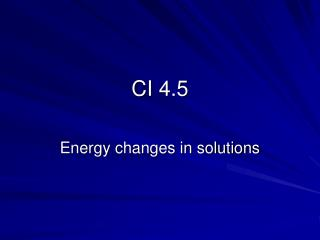DownloadDownload PresentationCI 4.5

# CI 4.5

Download Presentation## CI 4.5

- - - - - - - - - - - - - - - - - - - - - - - - - - - E N D - - - - - - - - - - - - - - - - - - - - - - - - - - -
##### Presentation Transcript

1. CI 4.5 Energy changes in solutions

2. Why do some ionic substances dissolve in water, whilst others are insoluble? • If there is enough energy to separate the ions in the lattice, the substance will be soluble Ions in solid lattice Ions in solution

3. -HLE is a positive value Lattice enthalpy, HLE • The enthalpy change when 1 mole of solid is formed from the separate ions • This is always an exothermic process • HLE is always negative • The energy to break up a lattice = - HLE

4. Which will give more negative HLE? • Small ionic charge • Large ionic charge • Small ionic radius • Large ionic radius    

5. 3- 3+ Where does the energy come from to break up the lattice? • Hydration

6. Exothermic – because bonds are made Is hydration exothermic or endothermic? Enthalpy of hydration,Hhyd • Hhyd is the enthalpy change when a solution of ions is made from 1 mole of gaseous ions

7. Example of hydration • Na+(g) + aq Na+(aq) • Hhyd = - 406 kJ mol-1

8. Different ions • An ionic compound contains cations and anions • Total Hhyd = Hhyd (cation) + Hhyd (anion) • When NaCl is dissolved: • Total Hhyd = Hhyd (Na+) + Hhyd (Cl-)

9. Which will give more negative Hhyd? • Small ionic charge • Large ionic charge • Small ionic radius • Large ionic radius    

10. Water is not the only solvent • Enthalpy of solvation, Hsolv , is used for other solvents

11. Enthalpy change of solution • This is the enthalpy change when 1 mole of a solute dissolves to form an infinitely dilute solution • Hsolution = Hhyd (cation) + Hhyd (anion) - HLE • If Hsolution is negative, it is more likely that the substance will dissolve

12. What decides solubility? • Energy is put in to separate the ions in the lattice [ - HLE] • Energy is released by hydration of the ions • If more energy is released than used up, then Hsolution will be negative and the solute is more likely to dissolve. [Hhyd (cation) + Hhyd (anion)]

13. Enthalpy cycle for solution Hsolution Ionic lattice + solvent solution - HLE Hhyd(cation) + Hhyd (anion) Gaseous ions + solvent Hsolution = Hhyd (cation) + Hhyd (anion) - HLE

14. Enthalpy level diagrams • Make it easier to compare the sizes of the enthalpy changes

15. Is ΔHsolution Endothermic or exothermic ? ΔHsolution = -ΔHLE + ΔHhyd(cat) + ΔHhyd(an) enthalpy Gaseous ions -ΔHLE ΔHhyd(cat) ΔHhyd(an) Solute + solvent ΔHsolution Solution

16. Is ΔHsolution exothermic or endothermic? ΔHsolution = -ΔHLE + ΔHhyd(cat) + ΔHhyd(an) enthalpy Gaseous ions ΔHhyd(cat) -ΔHLE ΔHhyd(an) Solution ΔHsolution Solute + solvent

17. Solubility • The more negative the value for ΔHsolution the more likely the solute is to dissolve. • If ΔHsolution is very large and positive, the solute will not dissolve. • If ΔHsolution is small and positive, the solute may dissolve, if there is sufficient increase in entropy.

18. Over to you! ? • Time to try the problems.